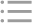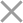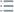## Fill out the form in 2 simple steps below:

Step 1

Step 2
** By clicking this button you agree to our Privacy Policy statementRequest our prices
--Select currency--
USD
EUR
* By clicking this button you agree to our Privacy Policy statementFree PVS‑Studio license for Microsoft MVP specialists
* By clicking this button you agree to our Privacy Policy statementTo get the licence for your open-source project, please fill out this form
* By clicking this button you agree to our Privacy Policy statementI am interested to try it on the platforms:
 Windows Linux macOS PVS-Studio for .NET Core JetBrains Rider
* By clicking this button you agree to our Privacy Policy statementMessage submitted.

Your message has been sent. We will email you at

check your Spam/Junk folder and click the "Not Spam" button for our message.
This way, you won't miss messages from our team in the future.

>
>
>
V636. Expression was implicitly cast fr…Introduction
Analyzing projects
On Windows
On Linux and macOS
Cross-platform
IDE
Build systems
Game Engines
Continuous use of the analyzer in software development
Deploying the analyzer in cloud Continuous Integration services
Managing analysis results
Analyzer diagnostics
General Analysis (C++)
General Analysis (C#)
General Analysis (Java)
Diagnosis of micro-optimizations (C++)
Diagnosis of micro-optimizations (C#)
Diagnosis of 64-bit errors (Viva64, C++)
Customer specific requests (C++)
MISRA errors
AUTOSAR errors
OWASP errors (C++)
OWASP errors (C#)
OWASP errors (Java)
Problems related to code analyzerContents

# V636. Expression was implicitly cast from integer type to real type. Consider using an explicit type cast to avoid overflow or loss of a fractional part.

Aug 27 2012

An expression contains a multiplication or division operation over integer data types. The resulting value is implicitly cast to a floating-point type. When detecting this, the analyzer warns you about a potential error that may cause an overflow or calculation of an incorrect result.

Below are examples of possible errors.

Case one. Overflow.

``````int LX = 1000;
int LY = 1000;
int LZ = 1000;
int Density = 10;
double Mass = LX * LY * LZ * Density;``````

We want to calculate an object's mass relying on its density and volume. We know that the resulting value may be a large one. That's why we declare the 'Mass' variable as the 'double' type. But this code doesn't take into account that there are variables of the 'int' type which are multiplied. As a result, we'll get an integer overflow in the right part of the expression and the result will be incorrect.

There are two ways to fix the issue. The first way is to change the variables' types:

``````double LX = 1000.0;
double LY = 1000.0;
double LZ = 1000.0;
double Density = 10.0;
double Mass = LX * LY * LZ * Density;``````

The second way is to use an explicit type conversion:

``````int LX = 1000;
int LY = 1000;
int LZ = 1000;
int Density = 10;
double Mass = (double)(LX) * LY * LZ * Density;``````

We can cast only the first variable to the 'double' type - that'll be enough. Since the multiplication operation refers to left-associative operators, calculation will be executed in the following way: (((double)(LX) * LY) * LZ) * Density. Consequently, each of the operands will be cast to the 'double' type before multiplication and we will get a correct result.

P.S. Let me remind you that it will be incorrect if you try to solve the issue in the following way: Mass = (double)(ConstMass) + LX * LY * LZ * Density. The expression to the right of the '=' operator will have the 'double' type, but it's still variables of the 'int' type that will be multiplied.

Case two. Loss of accuracy.

``````int totalTime = 1700;
int operationNum = 900;
double averageTime = totalTime / operationNum;``````

The programmer may be expecting that the 'averageTime' variable will have value '1.888(8)', but the result will equal '1.0' when executing the program. It happens because the division operation is performed over integer types and only then is cast to the floating-point type.

Like in the previous case, we may fix the error in two ways.

The first way is to change the variables' types:

``````double totalTime = 1700;
double operationNum = 900;
double averageTime = totalTime / operationNum;``````

The second way is to use an implicit type conversion.

``````int totalTime = 1700;
int operationNum = 900;
double averageTime = (double)(totalTime) / operationNum;``````

Note

Certainly, in some cases it's exactly division of integers that you need to execute. In such cases you can use the following comment to suppress false positives:

``//-V636``

This diagnostic is classified as:

 CWE-682CERT-FLP06-C
 You can look at examples of errors detected by the V636 diagnostic.